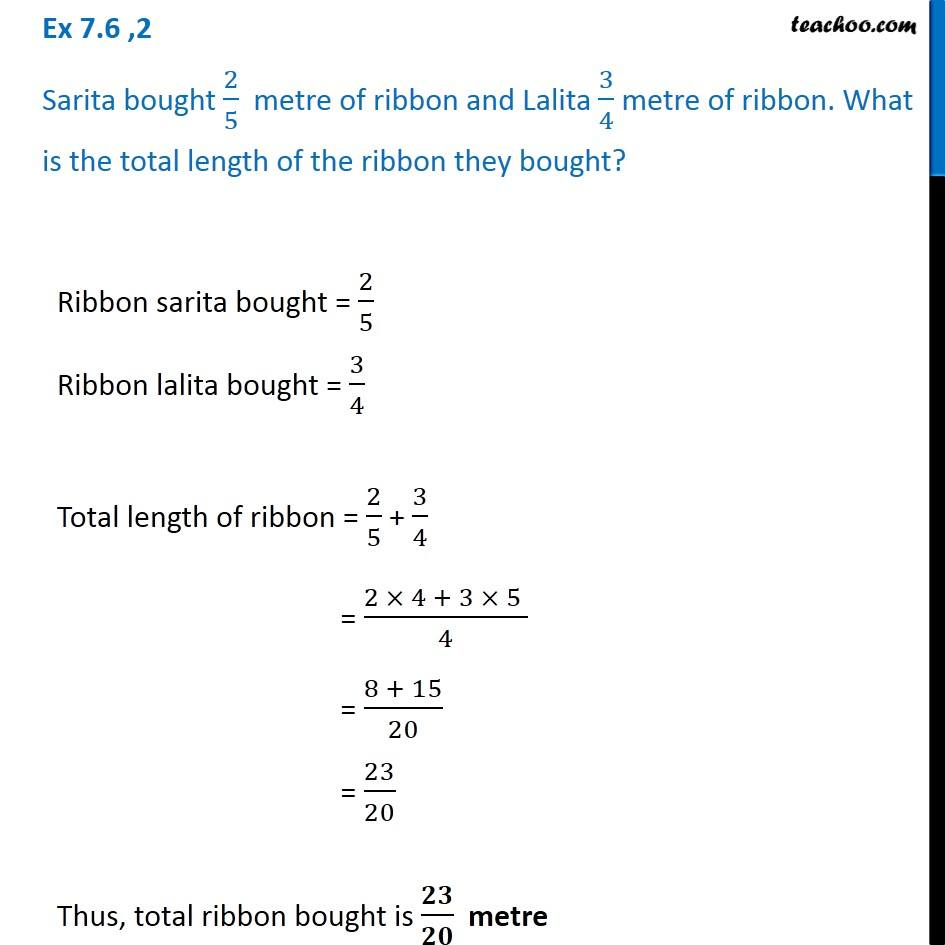1. Chapter 7 Class 6 Fractions
2. Serial order wise
3. Ex 7.6

Transcript

Ex 7.6 ,2 Sarita bought 2/5 metre of ribbon and Lalita 3/4 metre of ribbon. What is the total length of the ribbon they bought? Ribbon sarita bought = 2/5 Ribbon lalita bought = 3/4 Total length of ribbon = 2/5 + 3/4 = (2 4 + 3 5 )/4 = (8 + 15)/20 = 23/20 Thus, total ribbon bought is / metre

Ex 7.6

Chapter 7 Class 6 Fractions
Serial order wise